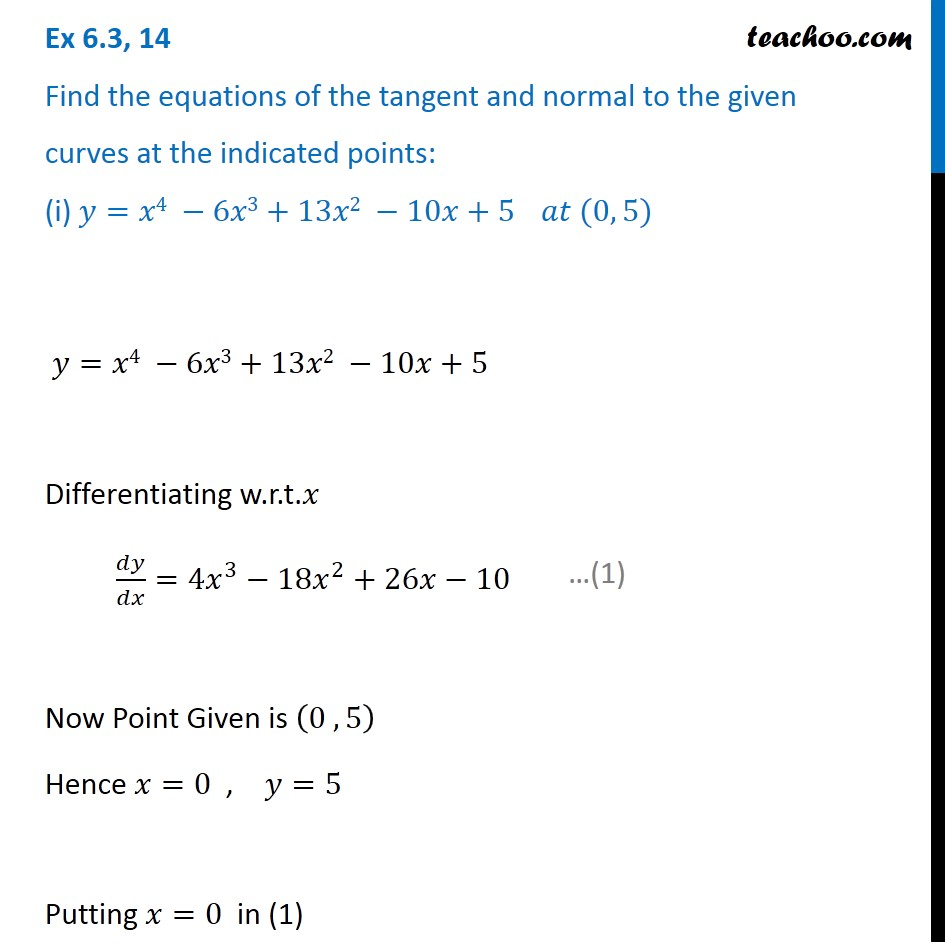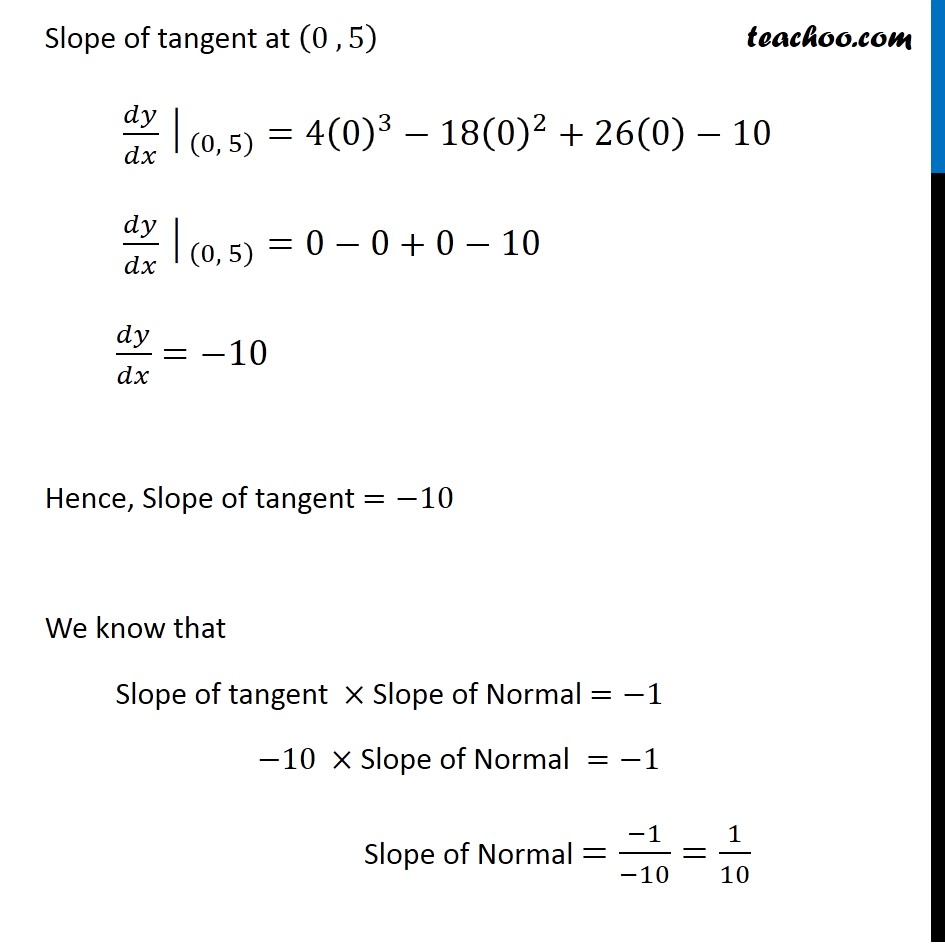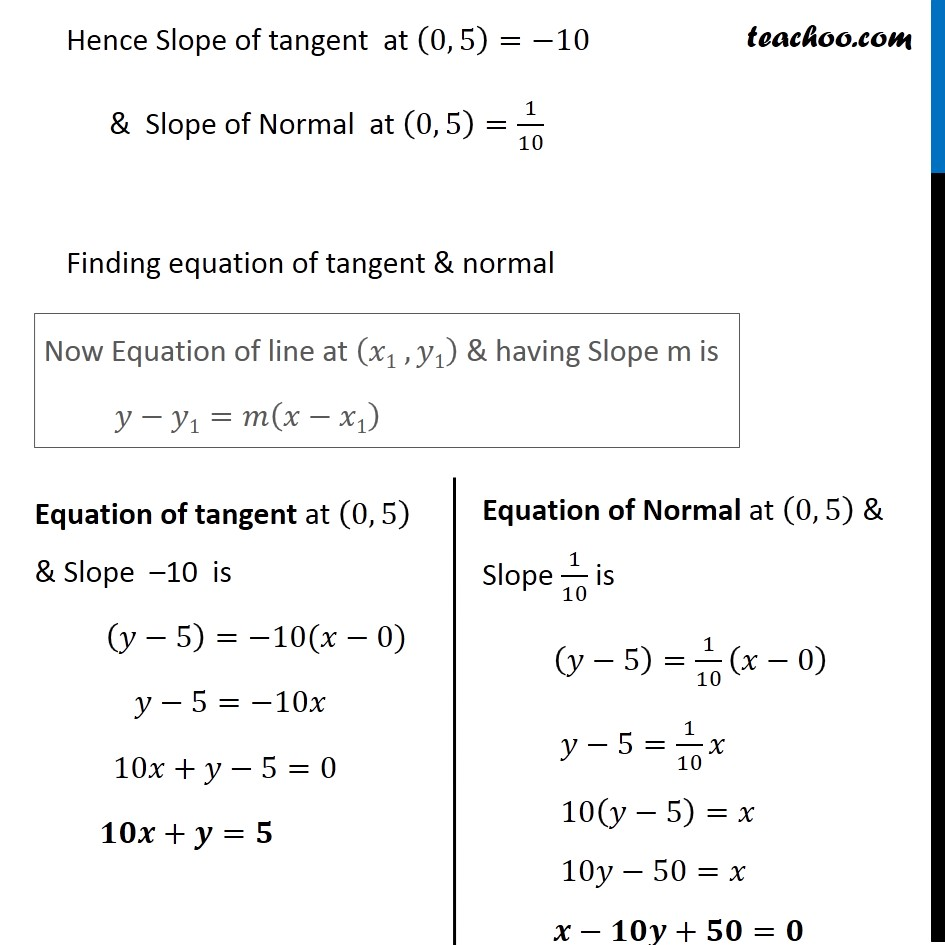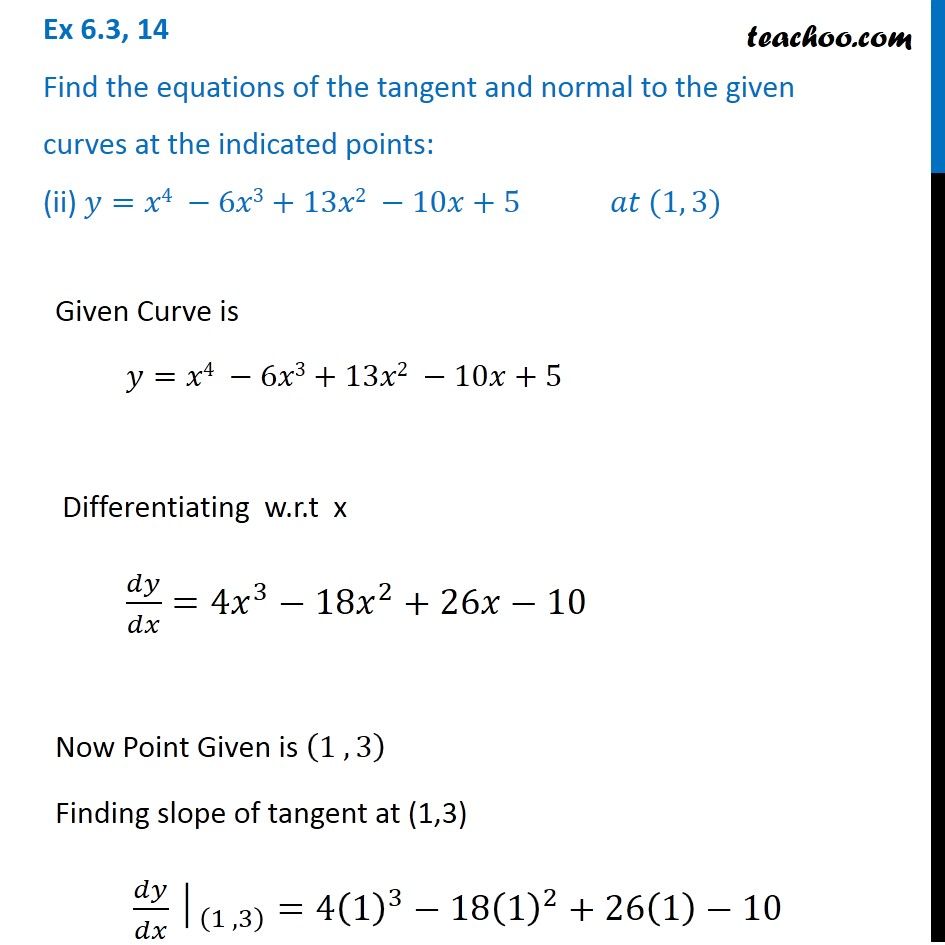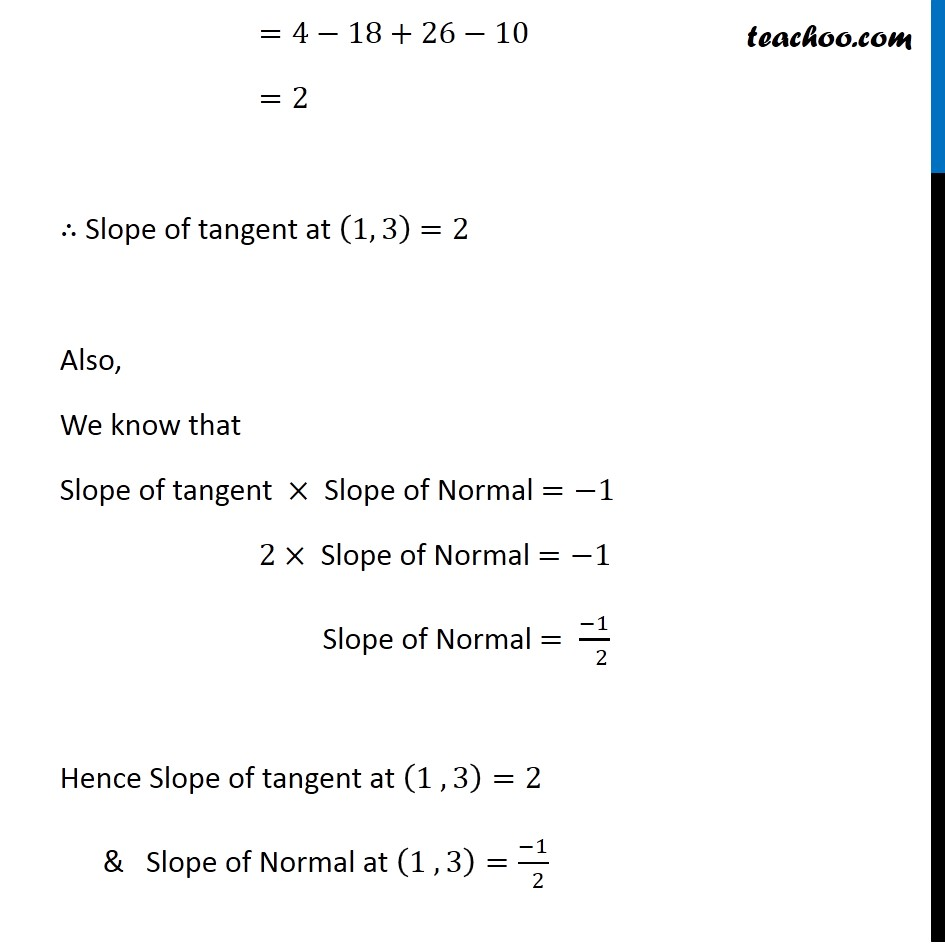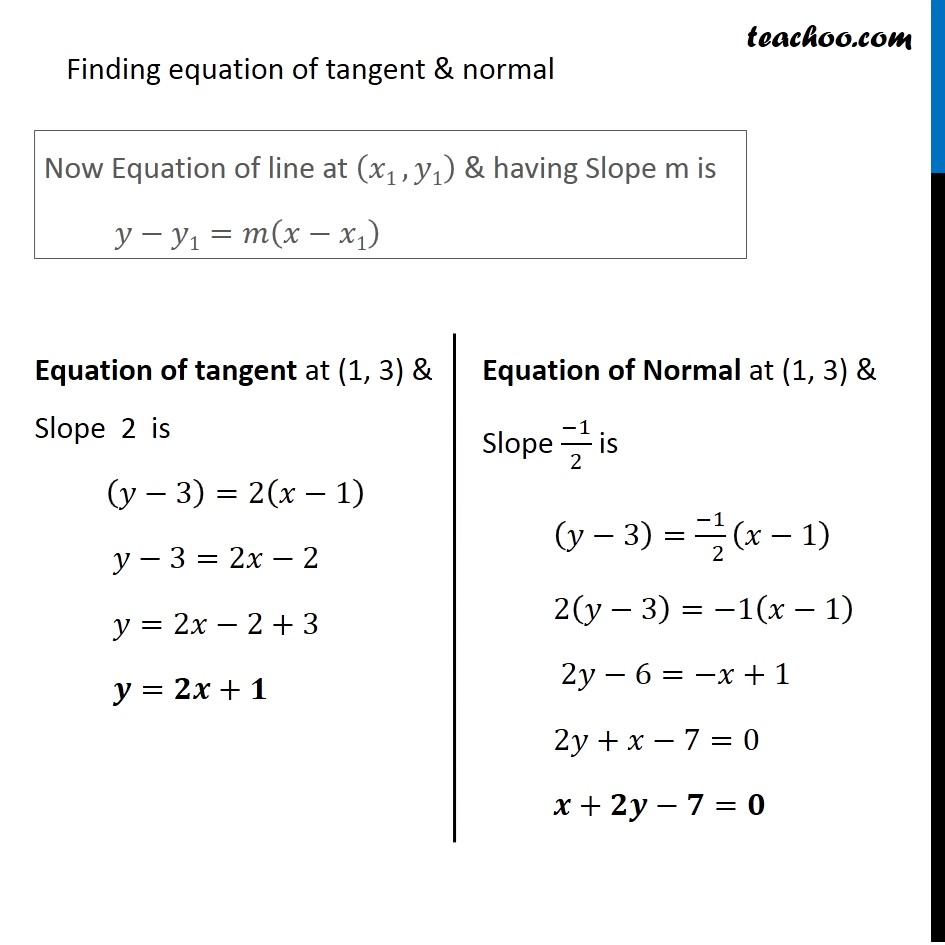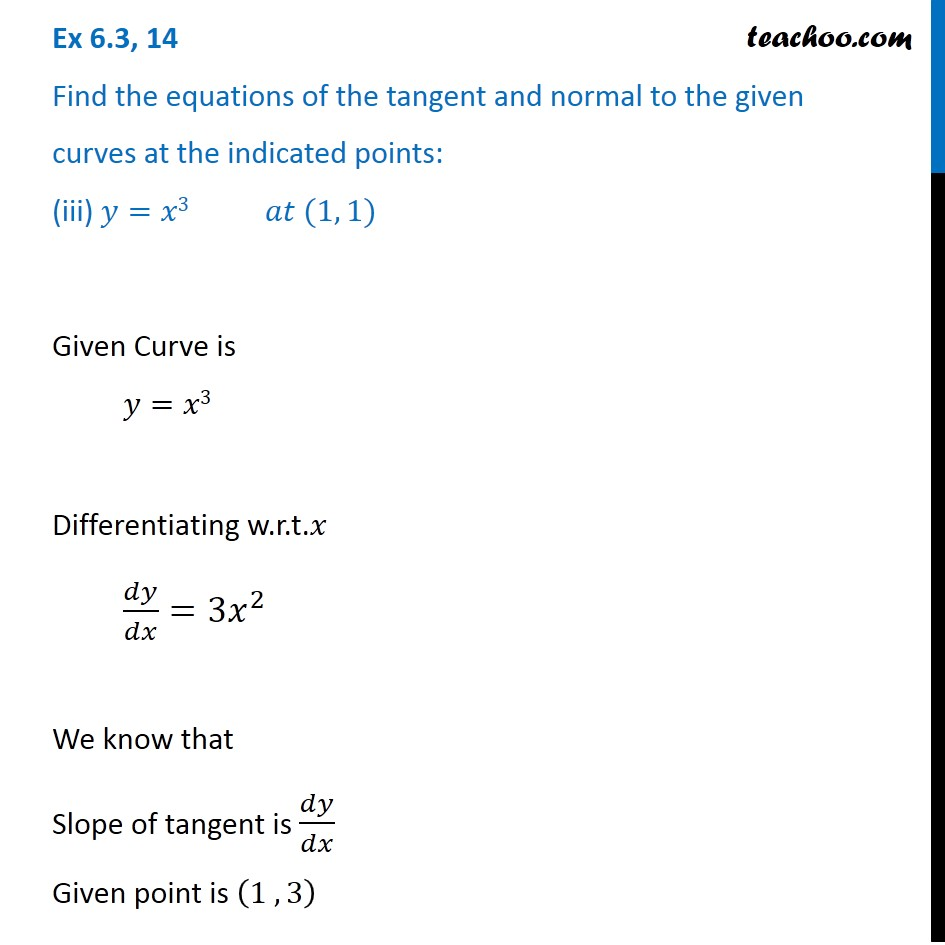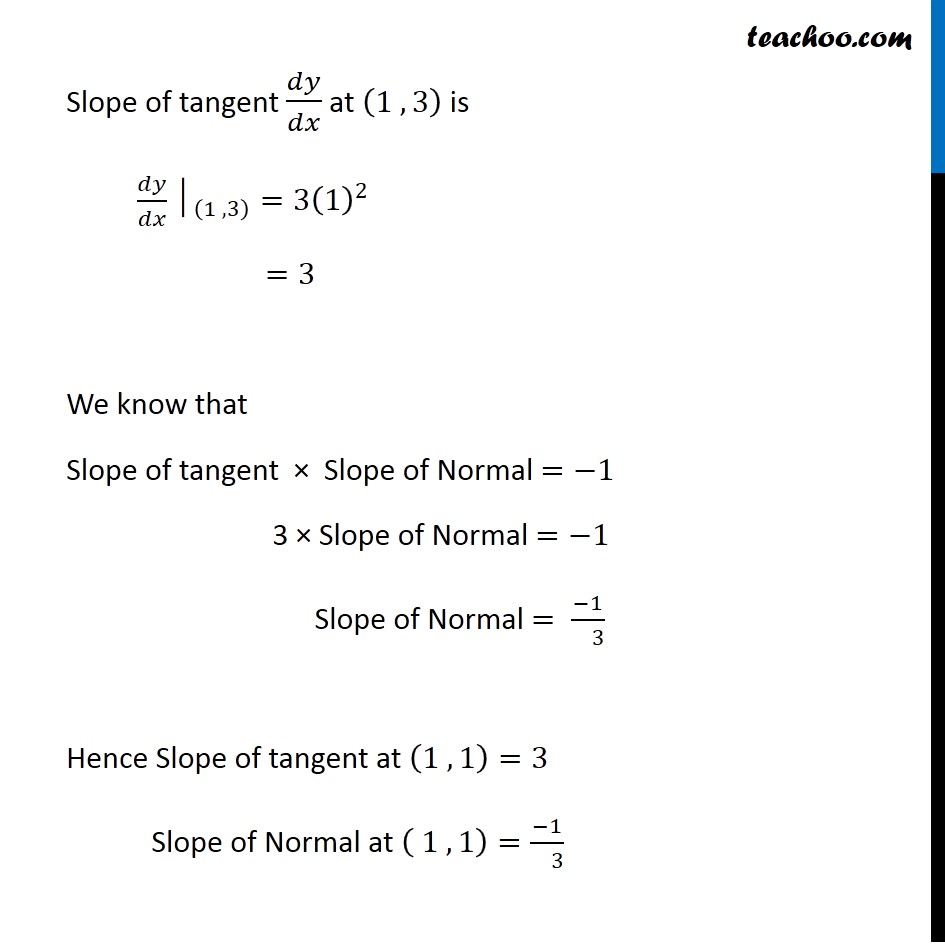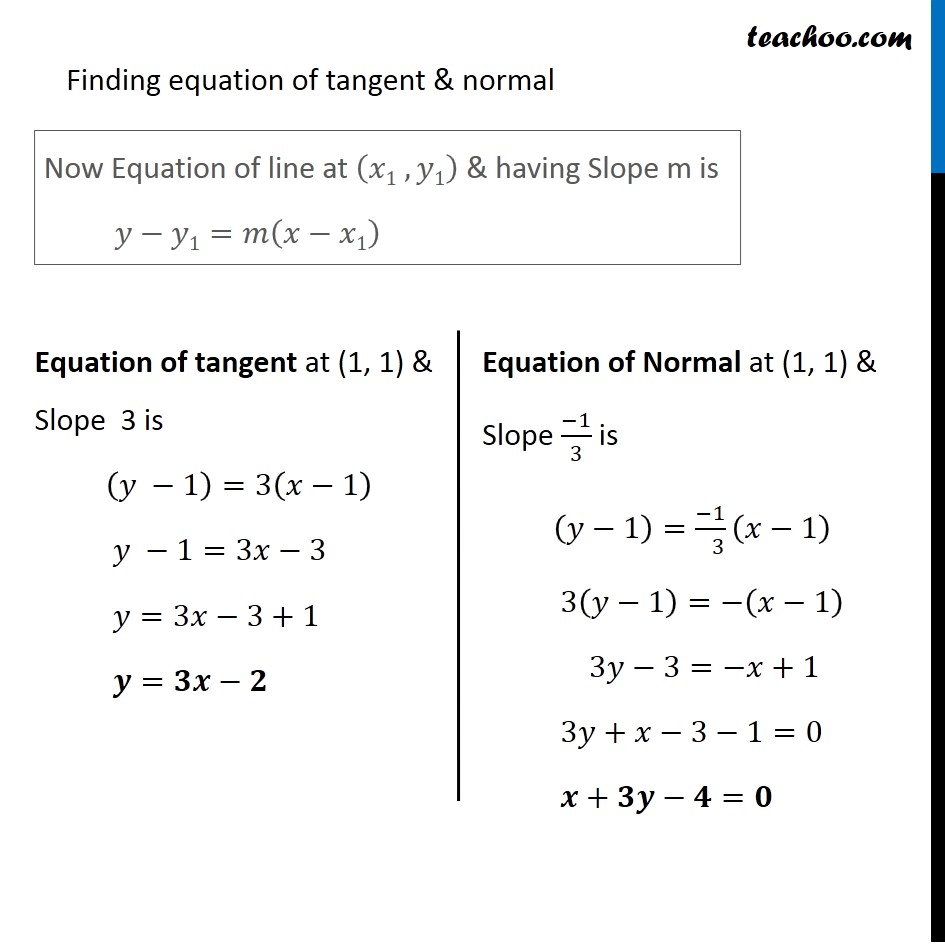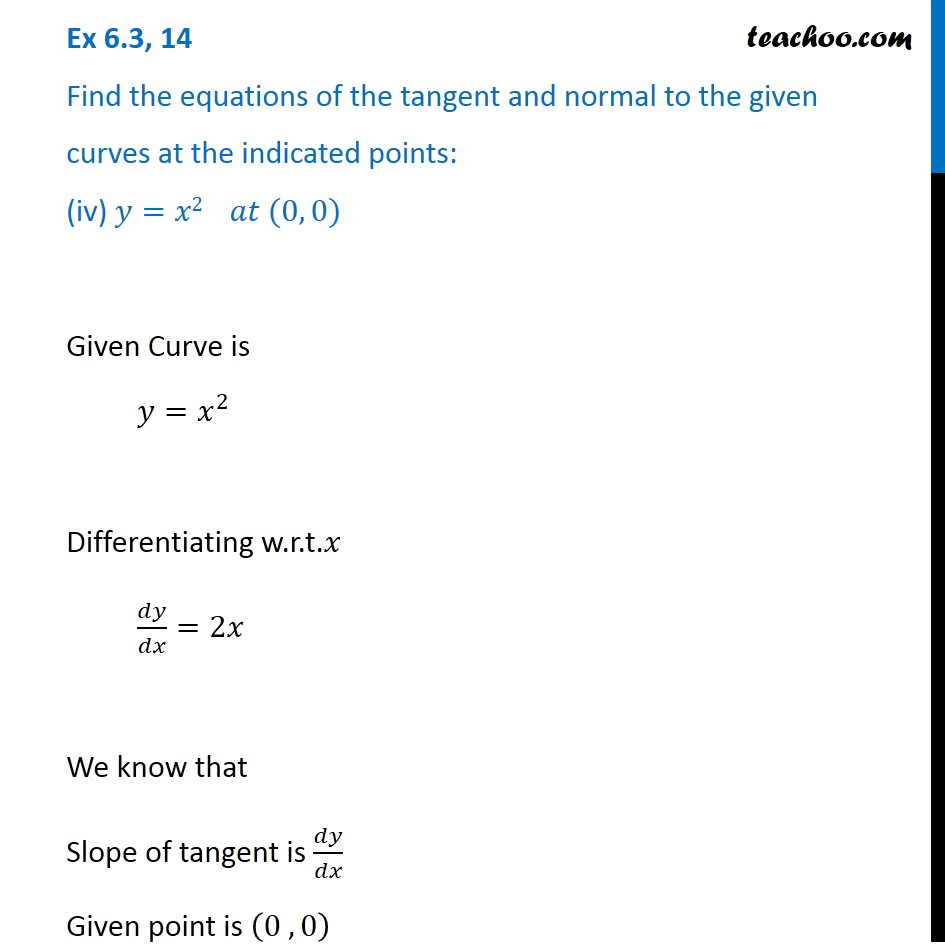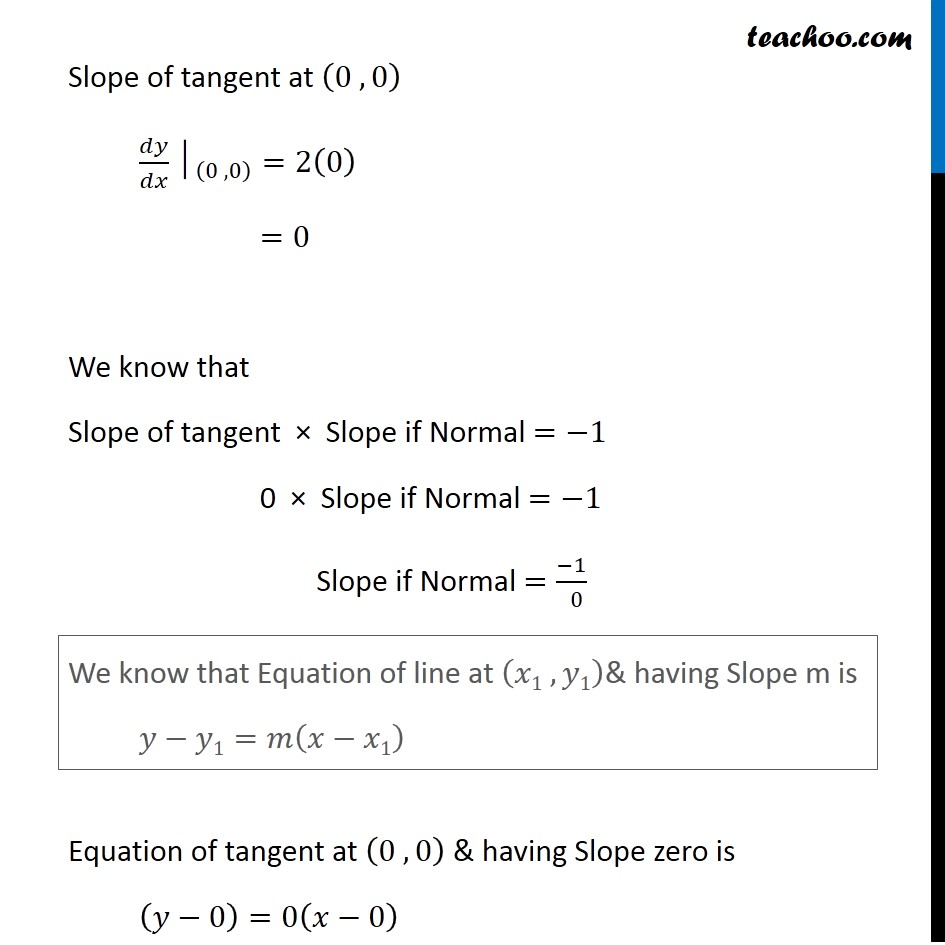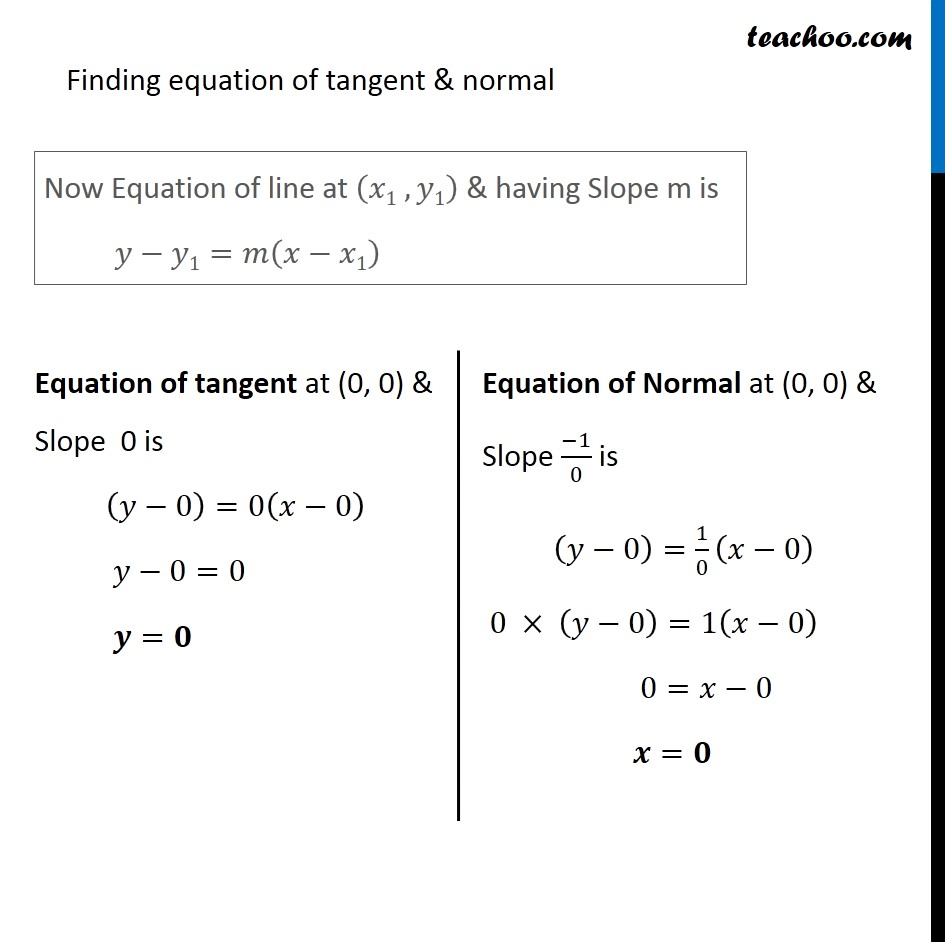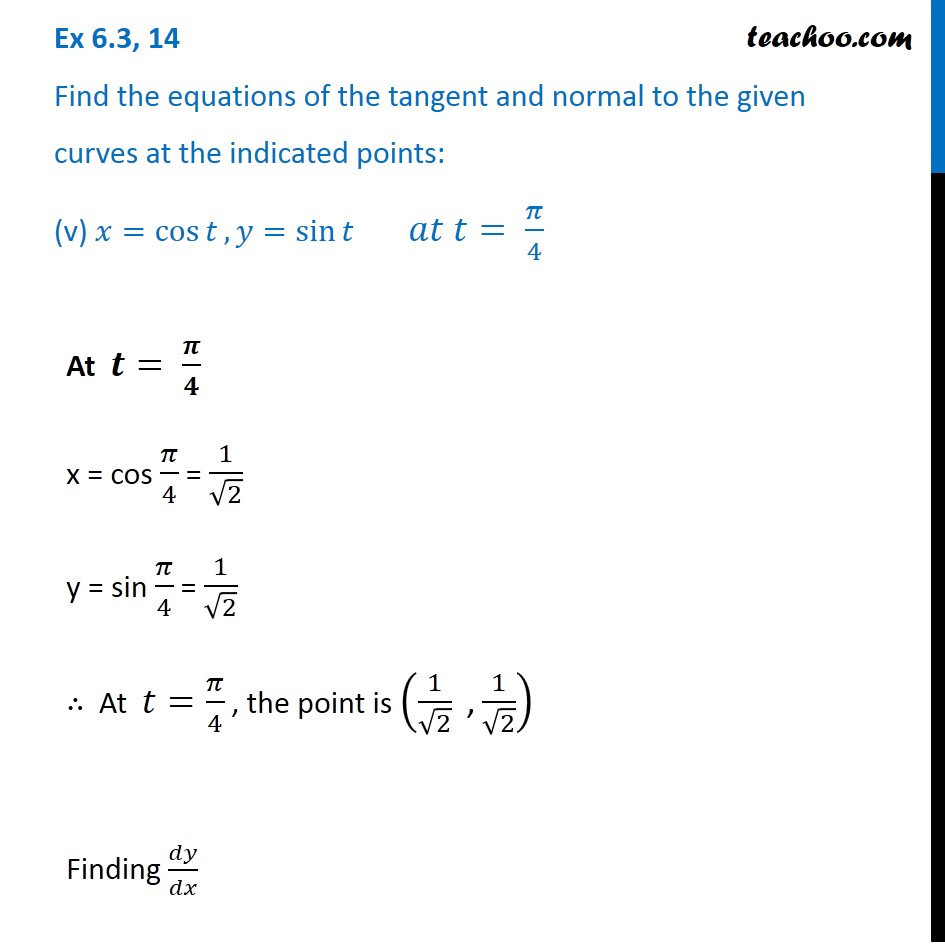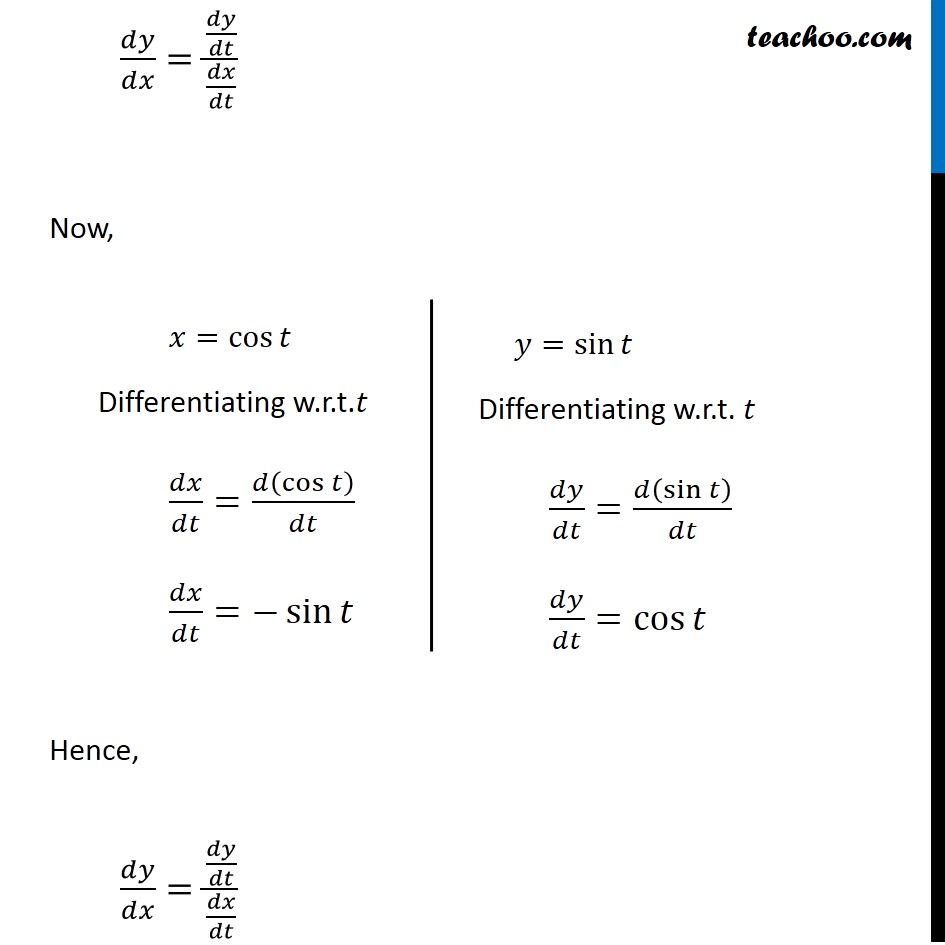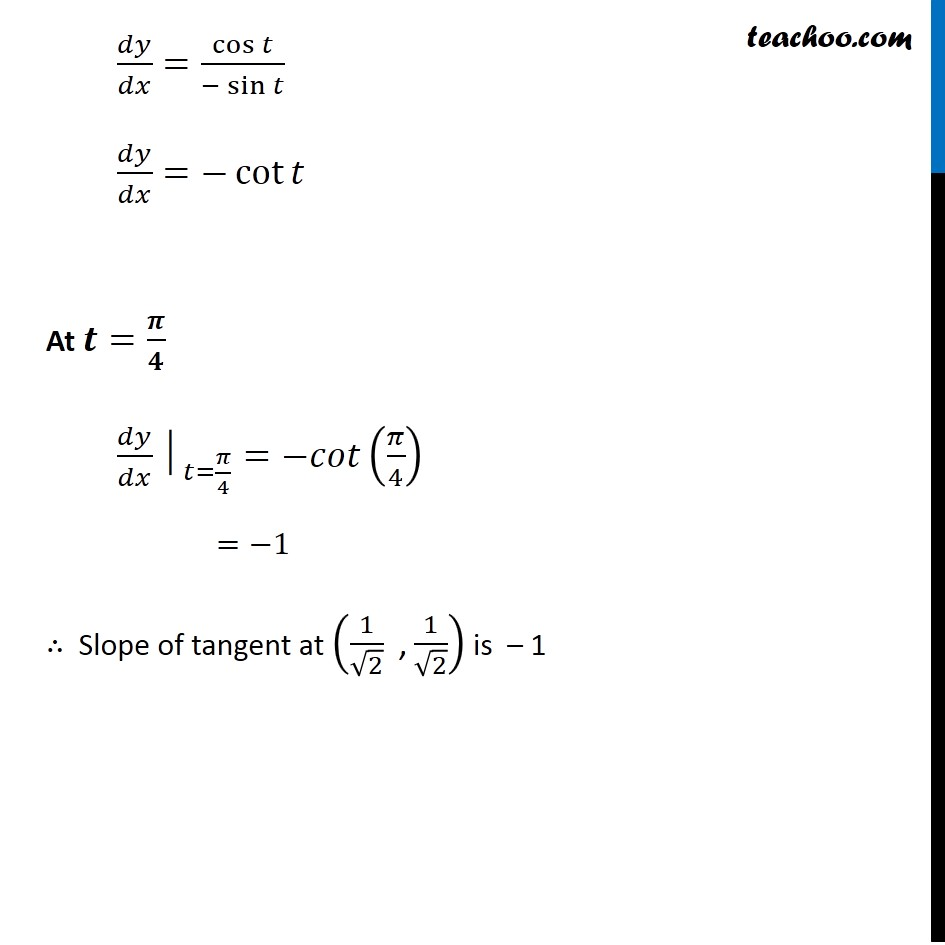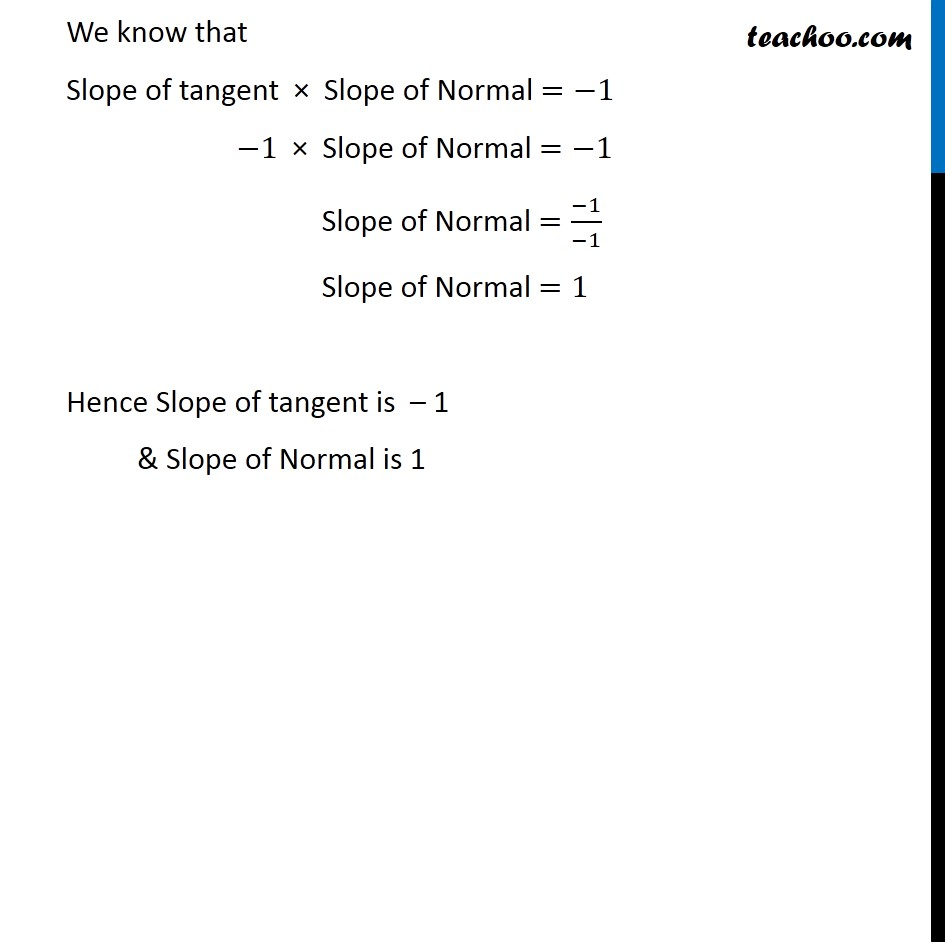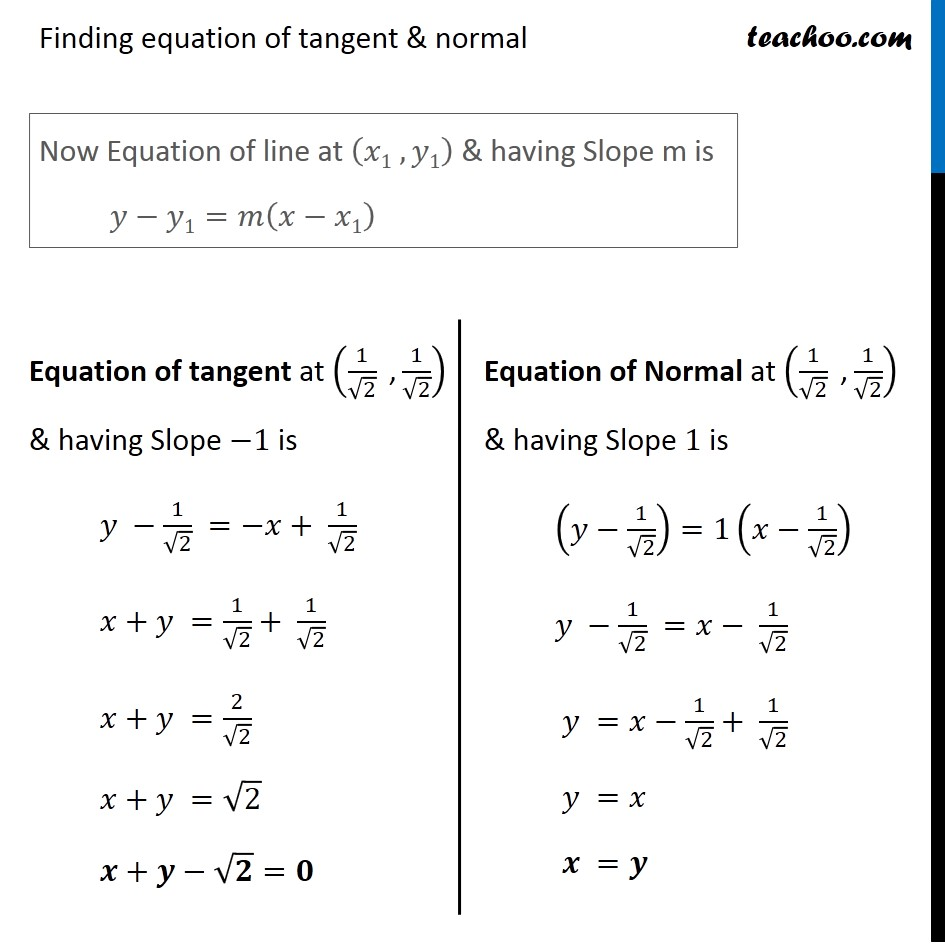Subscribe to our Youtube Channel - https://you.tube/teachoo

1. Chapter 6 Class 12 Application of Derivatives
2. Serial order wise
3. Ex 6.3

Transcript

Ex 6.3, 14 Find the equations of the tangent and normal to the given curves at the indicated points: (i) 𝑦=𝑥4 −6𝑥3+13𝑥2 −10𝑥+5 𝑎𝑡 (0, 5) 𝑦=𝑥4 −6𝑥3+13𝑥2 −10𝑥+5 Differentiating w.r.t. 𝑥 𝑑𝑦/𝑑𝑥=4𝑥^3−18𝑥^2+26𝑥−10 Now Point Given is (0 ,5) Hence 𝑥=0 , 𝑦=5 Putting 𝑥=0 in (1) Slope of tangent at (0 , 5) 〖𝑑𝑦/𝑑𝑥│〗_((0, 5) )=4(0)^3−18(0)^2+26(0)−10 〖𝑑𝑦/𝑑𝑥│〗_((0, 5) )=0−0+0−10 𝑑𝑦/𝑑𝑥=−10 Hence, Slope of tangent =−10 We know that Slope of tangent × Slope of Normal =−1 −10 ×"Slope of Normal "=−1 "Slope of Normal" =(−1)/(−10)=1/10 Hence Slope of tangent at (0, 5)=−10 & Slope of Normal at (0, 5)=1/10 Finding equation of tangent & normal Now Equation of line at (𝑥1 , 𝑦1) & having Slope m is 𝑦−𝑦1=𝑚(𝑥−𝑥1) Equation of tangent at (0, 5) & Slope –10 is (𝑦−5)=−10(𝑥−0) 𝑦−5=−10𝑥 10𝑥+𝑦−5=0 𝟏𝟎𝒙+𝒚=𝟓 Equation of Normal at (0, 5) & Slope 1/10 is (𝑦−5)=1/10 (𝑥−0) 𝑦−5=1/10 𝑥 10(𝑦−5)=𝑥 10𝑦−50=𝑥 𝒙−𝟏𝟎𝒚+𝟓𝟎=𝟎 Ex 6.3, 14 Find the equations of the tangent and normal to the given curves at the indicated points: (ii) 𝑦=𝑥4 −6𝑥3+13𝑥2 −10𝑥+5 𝑎𝑡 (1, 3) Given Curve is 𝑦=𝑥4 −6𝑥3+13𝑥2 −10𝑥+5 Differentiating w.r.t x 𝑑𝑦/𝑑𝑥=4𝑥^3−18𝑥^2+26𝑥−10 Now Point Given is (1 , 3) Finding slope of tangent at (1,3) 〖𝑑𝑦/𝑑𝑥│〗_((1 , 3) )=4(1)^3−18(1)^2+26(1)−10 =4−18+26−10 =2 ∴ Slope of tangent at (1, 3) =2 Also, We know that Slope of tangent × Slope of Normal =−1 2× Slope of Normal =−1 Slope of Normal = (−1)/( 2) Hence Slope of tangent at (1 , 3)=2 & Slope of Normal at (1 , 3)=(−1)/( 2) Finding equation of tangent & normal Now Equation of line at (𝑥1 , 𝑦1) & having Slope m is 𝑦−𝑦1=𝑚(𝑥−𝑥1) Equation of tangent at (1, 3) & Slope 2 is (𝑦−3)=2(𝑥−1) 𝑦−3=2𝑥−2 𝑦=2𝑥−2+3 𝒚=𝟐𝒙+𝟏 Equation of Normal at (1, 3) & Slope (−1)/2 is (𝑦−3)=(−1)/( 2) (𝑥−1) 2(𝑦−3)=−1(𝑥−1) 2𝑦−6=−𝑥+1 2𝑦+𝑥−7=0 𝒙+𝟐𝒚−𝟕=𝟎 Ex 6.3, 14 Find the equations of the tangent and normal to the given curves at the indicated points: (iii) 𝑦=𝑥3 𝑎𝑡 (1, 1) Given Curve is 𝑦=𝑥3 Differentiating w.r.t.𝑥 𝑑𝑦/𝑑𝑥=3𝑥^2 We know that Slope of tangent is 𝑑𝑦/𝑑𝑥 Given point is (1 , 3) Slope of tangent 𝑑𝑦/𝑑𝑥 at (1 , 3) is 〖𝑑𝑦/𝑑𝑥│〗_((1 , 3) )=3(1)^2 =3 We know that Slope of tangent × Slope of Normal =−1 3 × Slope of Normal =−1 Slope of Normal = (−1)/( 3) Hence Slope of tangent at (1 , 1)=3 Slope of Normal at ( 1 , 1)=(−1)/( 3) Finding equation of tangent & normal Now Equation of line at (𝑥1 , 𝑦1) & having Slope m is 𝑦−𝑦1=𝑚(𝑥−𝑥1) Equation of tangent at (1, 1) & Slope 3 is (𝑦 −1)=3(𝑥−1) 𝑦 −1=3𝑥−3 𝑦=3𝑥−3+1 𝒚=𝟑𝒙−𝟐 Equation of Normal at (1, 1) & Slope (−1)/3 is (𝑦−1)=(−1)/( 3) (𝑥−1) 3(𝑦−1)=−(𝑥−1) 3𝑦−3=−𝑥+1 3𝑦+𝑥−3−1=0 𝒙+𝟑𝒚−𝟒=𝟎 Ex 6.3, 14 Find the equations of the tangent and normal to the given curves at the indicated points: (iv) 𝑦=𝑥2 𝑎𝑡 (0, 0) Given Curve is 𝑦=𝑥^2 Differentiating w.r.t.𝑥 𝑑𝑦/𝑑𝑥=2𝑥 We know that Slope of tangent is 𝑑𝑦/𝑑𝑥 Given point is (0 , 0) Slope of tangent at (0 , 0) 〖𝑑𝑦/𝑑𝑥│〗_((0 , 0) )=2(0) =0 We know that Slope of tangent × Slope if Normal =−1 0 × Slope if Normal =−1 Slope if Normal =(−1)/( 0) Equation of tangent at (0 , 0) & having Slope zero is (𝑦−0)=0(𝑥−0) We know that Equation of line at (𝑥1 , 𝑦1)& having Slope m is 𝑦−𝑦1=𝑚(𝑥−𝑥1) Finding equation of tangent & normal Now Equation of line at (𝑥1 , 𝑦1) & having Slope m is 𝑦−𝑦1=𝑚(𝑥−𝑥1) Equation of tangent at (0, 0) & Slope 0 is (𝑦−0)=0(𝑥−0) 𝑦−0=0 𝒚=𝟎 Equation of Normal at (0, 0) & Slope (−1)/0 is (𝑦−0)=1/0 (𝑥−0) 0 × (𝑦−0)=1(𝑥−0) 0=𝑥−0 𝒙=𝟎 Ex 6.3, 14 Find the equations of the tangent and normal to the given curves at the indicated points: (v) 𝑥=cos⁡𝑡, 𝑦=sin⁡𝑡 𝑎𝑡 𝑡= 𝜋/4 At 𝒕= 𝝅/𝟒 x = cos 𝜋/4 = 1/√2 y = sin 𝜋/4 = 1/√2 ∴ At 𝑡=𝜋/4 , the point is (1/√2 " ," 1/√2) Finding 𝑑𝑦/𝑑𝑥 𝑑𝑦/𝑑𝑥=(𝑑𝑦/𝑑𝑡)/(𝑑𝑥/𝑑𝑡) Now, Hence, 𝑑𝑦/𝑑𝑥=(𝑑𝑦/𝑑𝑡)/(𝑑𝑥/𝑑𝑡) 𝑥=cos⁡𝑡 Differentiating w.r.t.𝑡 𝑑𝑥/𝑑𝑡=𝑑(cos⁡𝑡 )/𝑑𝑡 𝑑𝑥/𝑑𝑡=−sin⁡𝑡 𝑦=sin⁡𝑡 Differentiating w.r.t. 𝑡 𝑑𝑦/𝑑𝑡=𝑑(sin⁡𝑡 )/𝑑𝑡 𝑑𝑦/𝑑𝑡=cos⁡𝑡 𝑑𝑦/𝑑𝑥=cos⁡𝑡/(−sin⁡𝑡 ) 𝑑𝑦/𝑑𝑥=−cot⁡𝑡 At 𝒕=𝝅/𝟒 〖𝑑𝑦/𝑑𝑥│〗_(𝑡=𝜋/4)=−𝑐𝑜𝑡(𝜋/4) =−1 ∴ Slope of tangent at (1/√2 " ," 1/√2) is – 1 We know that Slope of tangent × Slope of Normal =−1 −1 × Slope of Normal =−1 Slope of Normal =(−1)/(−1) Slope of Normal =1 Hence Slope of tangent is – 1 & Slope of Normal is 1 Finding equation of tangent & normal Now Equation of line at (𝑥1 , 𝑦1) & having Slope m is 𝑦−𝑦1=𝑚(𝑥−𝑥1) Equation of tangent at (1/√2 " ," 1/√2) & having Slope −1 is 𝑦 −1/√2 =−𝑥+ 1/√2 𝑥+𝑦 =1/√2+ 1/√2 𝑥+𝑦 =2/√2 𝑥+𝑦 =√2 𝒙+𝒚−√𝟐=𝟎 Equation of Normal at (1/√2 " ," 1/√2) & having Slope 1 is (𝑦−1/√2)=1(𝑥−1/√2) 𝑦 −1/√2 =𝑥− 1/√2 𝑦 =𝑥−1/√2+ 1/√2 𝑦 =𝑥 𝒙 =𝒚

Ex 6.3